Next:4.7 To Probe FurtherUp:4.6 Amortized Algorithm AnalysisPrevious:4.6.4 Example of Incrementing Binary Integers

## 4.6.5 Amortized Analysis of Splaying

• As a measure of complexity, we shall take the depth within the tree that the desired node has before splaying.
•  T : BST on which we are performing a splay insertion or retrieval Ti : tree T as it has been transformed after step i of the splaying process, with T0 = T x : any node in Ti Ti(x) : subtree of Ti with root x | Ti(x)| : number of nodes of Ti(x)
• We consider bottom-up splaying at a node x, so that x begins somewhere in the tree T but after m splaying steps, ends up as the root of T.
• Rank

•

For each step i of the splaying process and each vertex x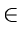T, we define rank at step i of x to be

ri(x) =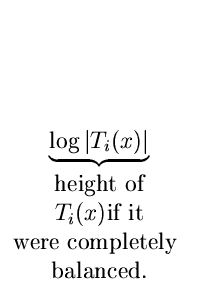• Portion out the credit balance of the tree among all its vertices by requiring the following credit invariant to hold:

•

For every node x of T and after every step i of splaying, node x has credit equal to its rank ri(x).

• Define the total credit balance for the tree as
Ci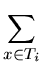ri(x)
• If the tree is empty or contains only one node, then its credit balance is 0. As the tree grows, its credit balance increases. The credit balance should reflect the work needed to build the tree.
• Our goal is to determine bounds on ai that will allow us to find the cost of the splaying process, amortized over a sequence of retrievals and insertions.
• A useful Result:

•

If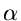,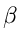,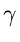are positive real numbers with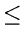, then
log+ log2log- 2                (*)

This can be easily proved as follows: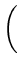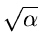-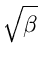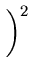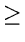0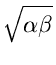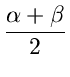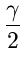log+ log2log- 2

Result 1

If the ith splaying step is a zig-zig or a zag-zag step at node x, then its amortized complexity ai satisfies

ai < 3(ri(x) - ri - 1(x))

See Figure 4.26.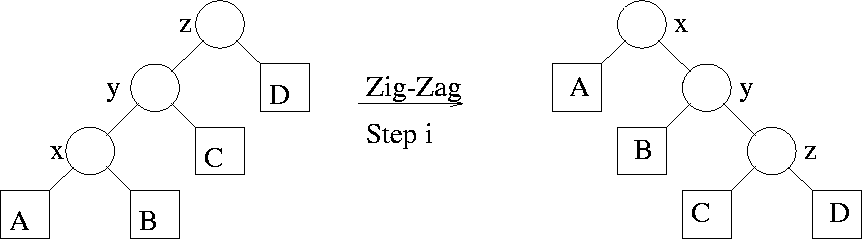• The actual complexity ti of a zig-zig or a zag-zag is 2 units
• Only the sizes of the subtrees rooted at x, y, z change in this step. Hence, all terms in the summation defining Ci cancel against those for Ci - 1 except those indicated below
•  ai = ti + Ci - Ci - 1 = 2 + ri(x) + ri(y) + ri(z) - ri - 1(x) - ri - 1(y) + ri - 1(z) = 2 + ri(y) + ri(z) - ri - 1(x) - ri - 1(y)                (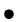)

Since | Ti(x)| = | Ti - 1(z)| as the subtree rooted at z before the splaying step has the same size as that rooted at x after the step.

Let= | Ti - 1(x)|,= | Ti(z)|, and= | Ti(x)|. Then observe that. This is because

 Ti - 1(x)  contains components  x, A, B Ti(z)  contains components z, C, D Ti(x)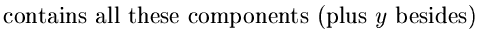.

Thus by (*

ri - 1(x) + ri(z2ri(x) - 22ri(x) - ri - 1(x) - ri(z) - 20
Adding this to (), we get
ai2ri(x) - 2ri - 1(x) + ri(y) - ri - 1(y)
Before step i, y is the parent of x so that
| Ti - 1(y)| > | Ti - 1(x)|
After step i, x is the parent of y so that
| Ti(x)| > | Ti(y)|
Taking logarithms we have
ri - 1(y) > ri - 1(x) and  ri(x) > ri(y)
Thus we obtain
ai < 3ri(x) - 3ri - 1(x)
Similarly, we can show Results 2 and 3 below:

Result 2

If the ith splaying step is a zig-zag or zag-zig at node x, then its amortized complexity ai satisfies

ai < 2(ri(x) - ri - 1(x))

Result 3

If the ith splaying step is a zig or a zag, then

ai < 1 + (ri(x) - ri - 1(x))

Result 4

The total amortized cost over all the splaying steps can be computed by adding the costs of all the splay steps. If there are k such steps, only the last can be a zig or zag and the others are all zig-zag or zig-zag or zag-zig or zag-zag. Since (*) provides the weaker bound, we get,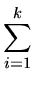ai =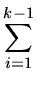ai + ak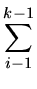3(ri(x) - ri - 1(x)) + (1 + 3rk(x) - 3rk - 1(x)) = 1 + 3rk(x) - 3r0(x)1 + 3rk(x) = 1 + 3log n

Thus the amortized cost of an insertion or retrieval with splaying in a BST with n nodes does not exceed 1 + 3log n upward moves of the target node in the tree.

Result 5

Now consider a long sequence of m splay insertions or retrievals. We seek to compute the actual cost of all these m operations.

Let Tj and Aj be the actual cost and amortized cost of the jthoperation, where j = 1, 2,..., m, now represents a typical insertion or retrieval operation.

We have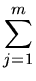Tj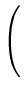Aj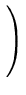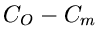If the tree never has more than n nodes, then C0 and Cm lie between 0 and log n, so that

C0 - Cmlog n
Also we know that

Aj1 + 3logn  for j = 1, 2,..., m
Thus the above becomesTj = m(1 + 3logn) + log n
The above gives the total actual time of m insertions or retrievals on a tree that never has more than n nodes.

This gives O(log n) actual running time for each operation.Next:4.7 To Probe FurtherUp:4.6 Amortized Algorithm AnalysisPrevious:4.6.4 Example of Incrementing Binary Integers
eEL,CSA_Dept,IISc,Bangalore